# TOPIC 7: MATRICES AND TRANSFORMATION ~ MATHEMATICS FORM 4### TOPIC 7: MATRICES AND TRANSFORMATION ~ MATHEMATICS FORM 4

Operations on Matrices

The Concept of a Matrix
Explain the concept of a matrix
Definition:
A matrix is an array or an Orderly arrangement of objects in rows and columns.
Each object in the matrix is called an element (entity).
Consider the following table showing the number of students in each stream in each form.
 Form I II III IV Stream A 38 35 40 28 Stream B 36 40 34 39 Stream C 40 37 36 35
From the above table, if we enclose the numbers in brackets without changing
their arrangement, then a matrix is farmed, this can be done by
removing the headings and the bracket enclosing the numbers (elements)
and given a name (normally a capital letter).
Nowthe above information can be presented in a matrix form as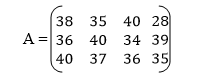Any matrix has rows and columns but sometimes you may find a matrix with only row without Colum or only column without row.
In the matrix A above, the numbers 38, 36 an 40 form the first column and 38, 35, 40 and 28 form the first row.
Matrix A above has three (3) rows and four (4) columns.
In
the matrix A, 34 is the element (entity) in the second row and third
column while 28 lies in the first row and fourth column. The plural form
of matrix is matrices.
Normallymatrices are named by capital letters and their elements by small letters which represent real numbers.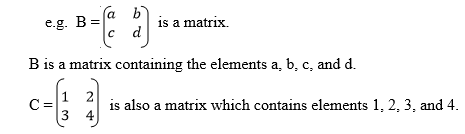Order of a matrix (size of matrix)
The order of a matrix or size of a matrix is given by the number of its rows and the number of its columns.
So if A has m rows and n columns, then the order of matrix is m x n.
It
is important to note that the order of any matrix is given by stating
the number of its rows first and then the number of its columns.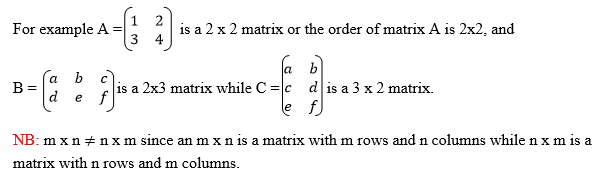Types of matrices:
The following are the common types of matrices:-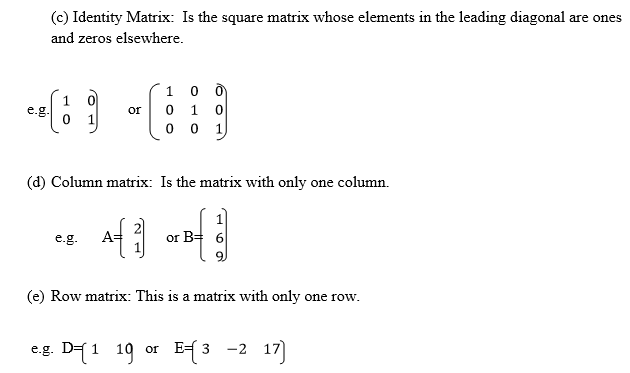Matrices of order up to 2 X 2
Add matrices of order up to 2 X 2
When adding or subtracting one matrix from another, the corresponding elements (entities) are /added or subtracted respectively.
This being the case, we can only perform addition and subtraction of matrices with the same orders.
Example 1
Given that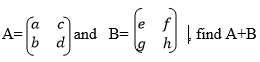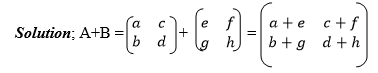Matrices of order up to 2 X 2
Subtract matrices of order up to 2 X 2
Example 2
Given that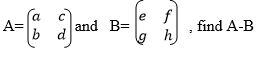Example 3
Solve for x, y and z in the following matrix equation;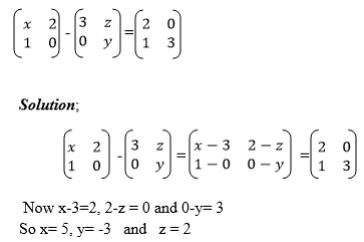Exercise 1
Determine the order of each of the following matrices;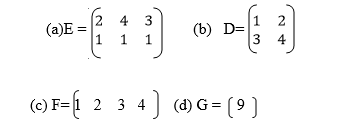2. Given that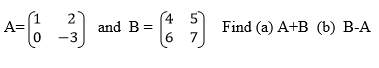3. Given that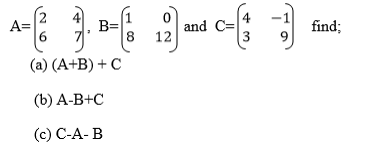4.
A house wife makes the following purchases during one week: Monday 2kg
of meat and loaf of bread Wednesday, 1kg of meat and Saturday, 1kg of
meat and one loaf of bread. The prices are 6000/= per kg of meat and
500/= per loaf of bread on each purchasing day
1. Write a 3×2 matrix of the quantities of items purchased over the three days .
2. Write a 2×1 column matrix of the unit prices of meat and bread.
5. Solve for x, y and z in the equation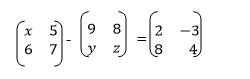If M is any square matrix, that is a matrix with order mxm or nxn and Z is another matrix with the same order as m such that
M+ Z= Z+M = M then Z is the additive identity matrix.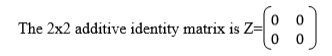The additive inverse of a matrix.
If
A and B are any matrices with the same order such that A+B = Z, then it
means that either A is an additive inverse of B or B is an additive
inverse of A that is B=-A or A= -B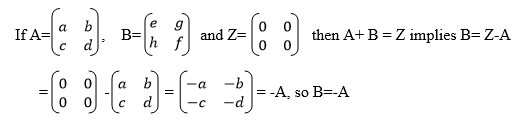Example 4
Find the additive inverse of A,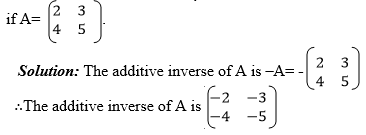Example 5
Find the additive identity of B if B is a 3×3 matrix.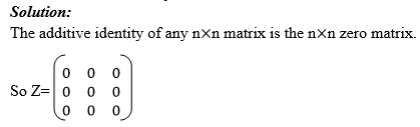A Matrix of Order 2 X 2 by a Scalar
Multiply a matrix of order 2 X 2 by a scalar
A matrix can be multiplied by a constant number (scalar) or by another matrix.
Scalar multiplication of matrices:
Rule: If A is a matrix with elements say a, b, c and d, or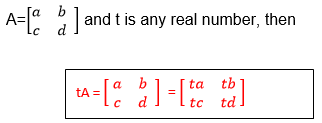Example 6
Given that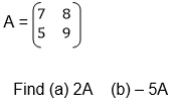Solution;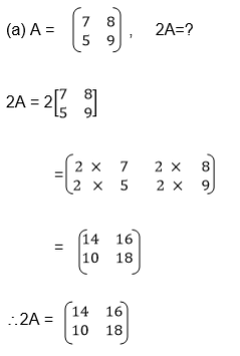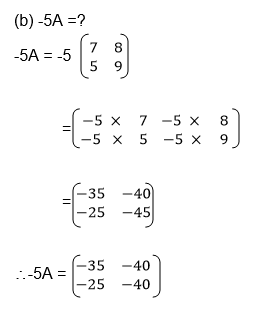Example 7
Given,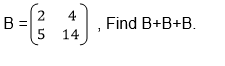Solution;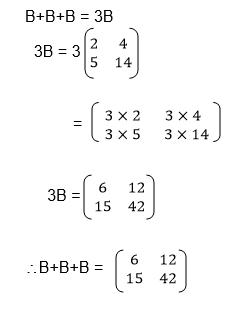Two Matrices of order up to 2 X 2
Multiply two matrices of order up to 2 X 2
Multiplication of Matrix by another matrix: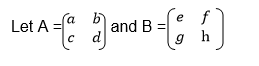AB is the product of matrices A and B while BA is the product of matrix B and A.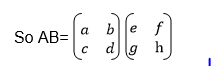In
AB, matrix A is called a pre-multiplier because it comes first while
matrix B is called the post multiplier because it comes after matrix A.
Rules of finding the product of matrices;
1. The pre –multiplier matrix is divided row wise, that is it is divided according to its rows.
2. The post multiplier is divided according to its columns.
3. Multiplication is done by taking an element from the row and multiplied by an element from the column.
4. In
rule (iii) above, the left most element of the row is multiplied by the
top most element of the column and the right most element from the row
is multiplied by the bottom most element of the column and their sums
are taken: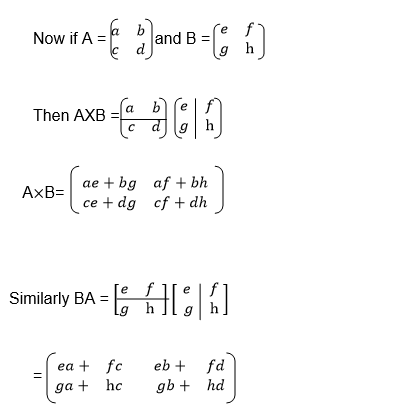Therefore
it can be concluded that matrix by matrix multiplication is only
possible if the number of columns in the pre-multiplier is equal to the
number of rows in the post multiplier.
Example 8
Given That;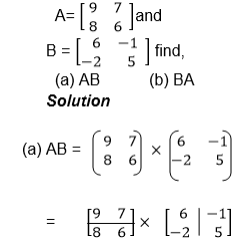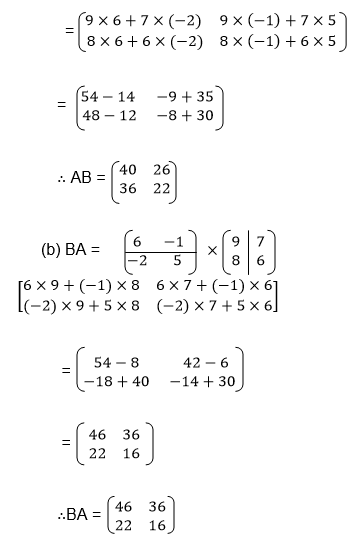From
the above example it can be noted that AB≠BA, therefore matrix by
matrix multiplication does not obey commutative property except when the
multiplication involves and identity matrix i.e. AI=IA=A
Example 9
Let,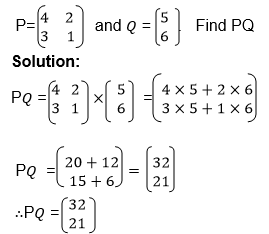Example 10
Find C×D if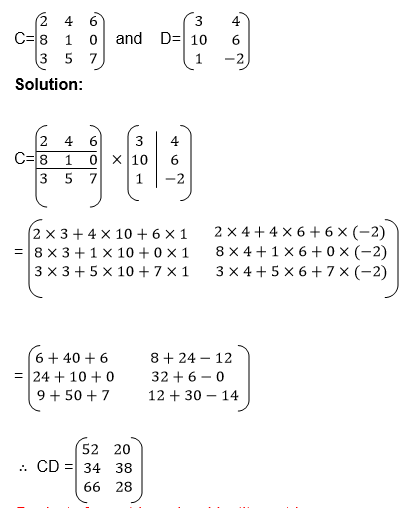Product of a matrix and an identity matrix:
If A is any square matrix and I is an identity matrix with the same order as A, then AI=IA=A
Example 11
Given;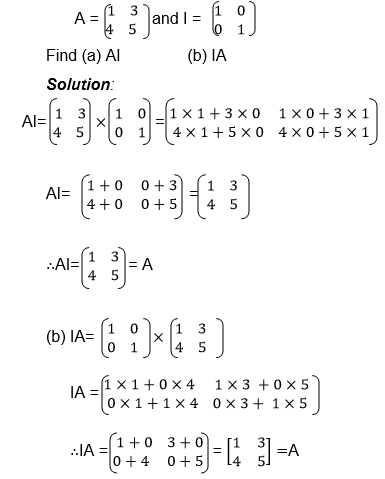Exercise 2
1. Given that A= (3 4) and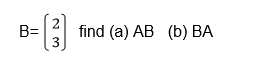2. If,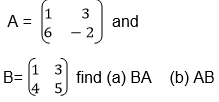3.Using the matrices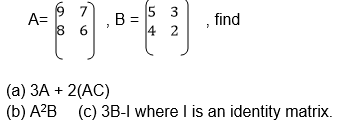4.Find the values of x and y if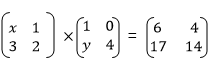Definition:
A transformation in a plane is a mapping which moves an object from one
position to another within the plane. Figures on the plane can also be
shifted from one position by a transformation.
A new position after a transformation on is called the image.
Examples of transformations are (i) Reflection (ii) Rotation (iii) Enlargement (iv) Translation.
Any Point P(X, Y) into P¹(X¹,Y¹) by Pre-Multiplying (ᵡᵧ) with a Transformation Matrix T
Transform any point P(X, Y) into P¹(X¹,Y¹) by pre-multiplying (ᵡᵧ) with a transformation matrix T
– Suppose a point P(x,y) in the x-y plane moves to a point P¢ (x¢,y¢) by a transformation T,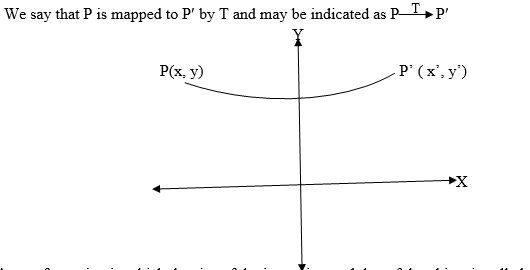A transformation in which the size of the image is equal that of the object is called an ISOMETRIC MAPPING.
The Matrix to Reflect a Point P(X, Y ) in the X-Axis
Apply the matrix to reflect a point P(X, Y ) in the x-axis
Reflection;
When
you look at yourself in a mirror you seem to see your body behind the
mirror. Your body is in front of the mirror as your image is behind it.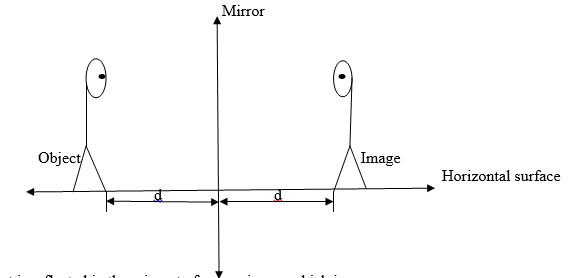An object is reflected in the mirror to form an image which is;
1. The same size as the object
2. The same distance from the mirror as the object
So reflection is an example of ISOMETRIC MAPPING.
The mirror is the line of symmetry between the object and the image.
Example 24
Find the image of the point A (2,3) after reflection in the x – axes.
Solution;
Plot point A and its image A¢ such that AA¢ crosses the x – axis at B and also perpendicular to it.
For reflection AB should be the same as BA¢ i.e. AB = BA¢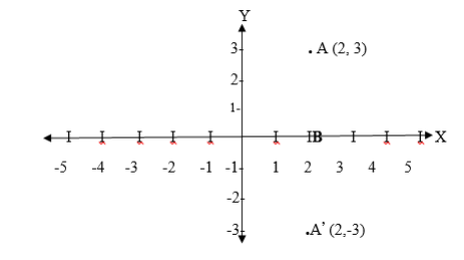From the figure, the coordinates of A ¢ are A¢ (2,-3). So the image of A (2,3) under reflection in the x-axis is A¢ (2,-3)
Normally the letter M is used to denote reflection and thus Mx means reflection in the x – axis.
So Mx(2,3) =- (2,-3).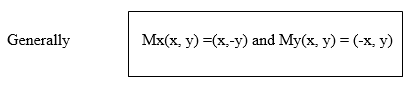Where Mx means reflection in the x – axis and My means reflection in the y-axis.
The Matrix to Reflect a Point P(X, Y) in the Y-Axis
Apply the matrix to reflect a point P(X, Y) in the Y-Axis
Example 25
Find the image of B(3,4) under reflection in the y- axis.
Solution:
From My (x.y)= (-x,y)
My (3 ,4 ) =( -3,4)
Therefore the image of B(3,4) is B'(-3,4) .
Reflection in the line y = x.
The line y=x makes an angle 45with
x and y axes. It is the line of symmetry for the angle YOX formed by
two axis. By using isosceles triangle properties, reflection of the
point (1,0) in the line y=x will be ( 0,1) while the reflection of (0,2)
in the line y=x will be ( 2, 0) it can be noticed that the coordinates
are exchanging positions. Hence the reflection of the point (x,y) in the
line y=x is ( y,x).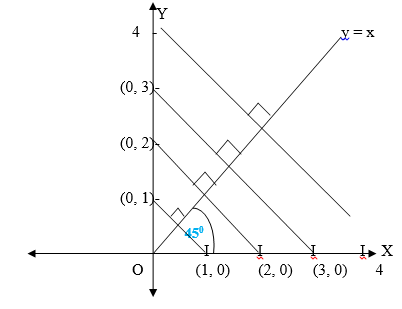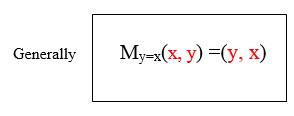Where My =xmeans reflection in the line y=x.
Example 26
Find the image of the point A(1,2) after reflection in the line y = x . Draw a sketch.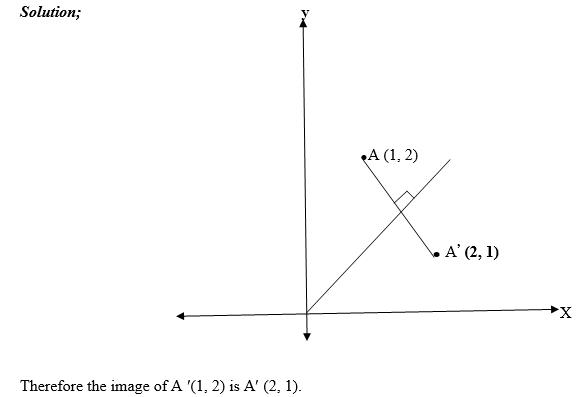Reflection in the line y = -x
The reflection of the point B(x,y) in the line y = -x is B'(-y,-x).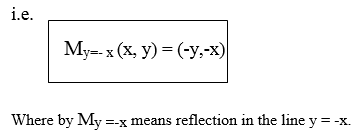Example 27
Find the image of B (3,4) after reflection in the line y=-x followed by another reflection in the line y=0.Draw a sketch.
Solution;
Reflection of B in the line y=-x is B'(-4,-3). The line y=0 is the x – axis. So reflection (-4,-3) in the x-axis is (-4,3)
Therefore the image of B (3,4) is B¢(-4,3).
The image of a point P(x,y) when reflected in the line making an angleαwith positive x-axis and passing through the origin.
If
the line passes through the origin and makes an angle a with x – axis
in the positive direction, then its equation is y= xtanα where tanαis
the slope of the line.
Consider the following diagram.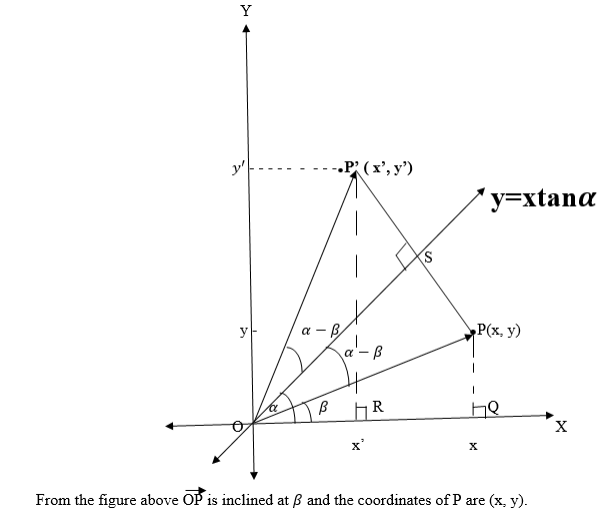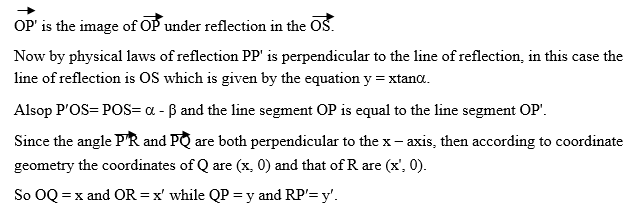But OPQ is a right angled triangle.
So x = OP Cosβ and y = OPSinβ .
Again
OP¢R is a right angled triangle and the angle P¢QR = a -β + a- β+ β,
this is due to the fact that reflection is an isometric mapping.
Now the angle P¢OR = 2 a-β, then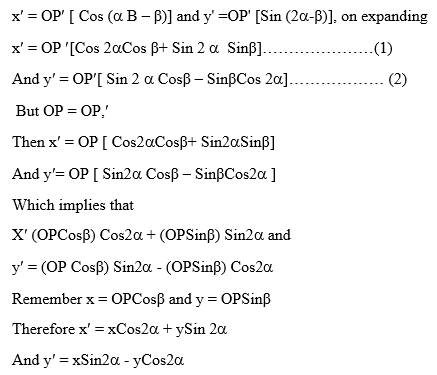It follows therefore that if M is a reflection in the line inclined at a, then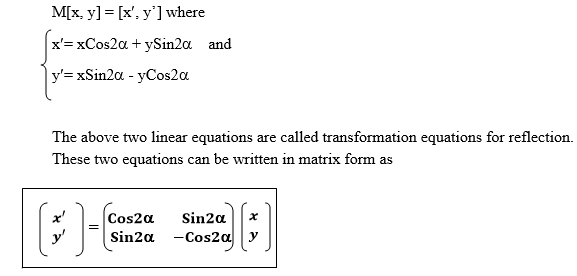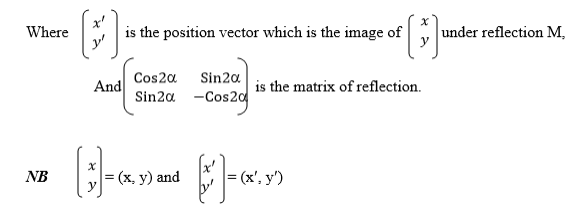Example 28
Find the image of the point A (1, 2) after a reflection in the line y = x.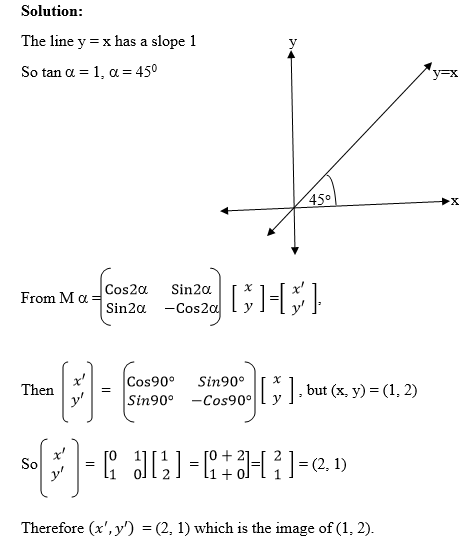Example 29
Find the image of B (3,4) after reflection in the line y = -x followed by another reflection in the line y = 0.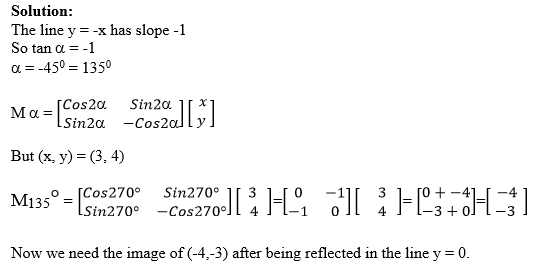But the line y = 0 has 0 slope because it is the x – axis,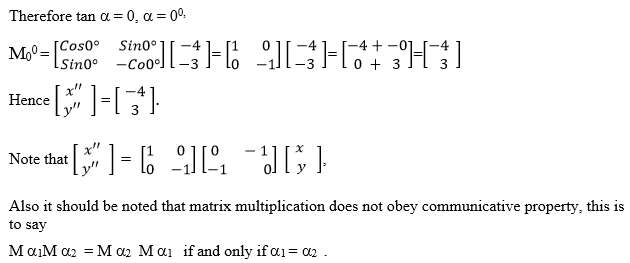Example 30
Find the equation of the line y = 2x + 5 after being reflected in the line y = x,
Solution:
The line y = x has a slope 1
So tan a = 1 which means a = 450
To
find the image of the line y = 2x + 5, we choose at least two points on
it and find their images, then we use the image points to find the
equation of the image line.
Now y = 2x + 5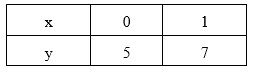The points (0,5) and (1,7) lie on the line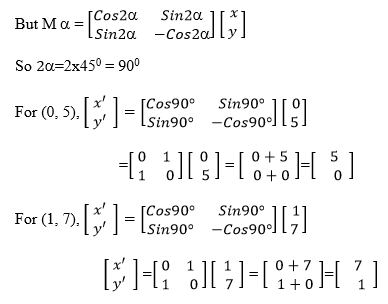So the image line is the line passing through (5,0) and (7,1) and it is obtained as follows;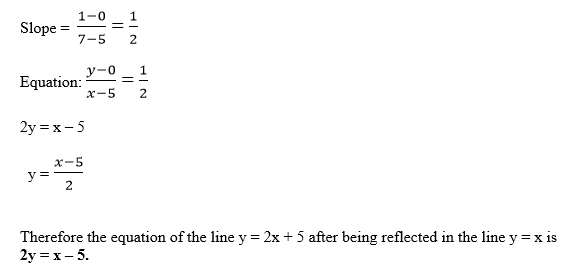Exercise 5
Self Practice.
1. Find the image of the point D (4,2) under reflection in the x – axis
2. Point Q (-4,3) is reflected in the y – axis. Find its image coordinates.
3. Reflect the point (5,4) in the line y = x
4. Find the image of the point (1,2) after a reflection in the line y = x followed by another reflection in the line y = -x.
5. Find the equation of the line y = 3x -1 after being reflected in the line x + y = 0.
A Matrix Operator to Rotate any Point P( X, Y ) Through 90° 180°, 270° and 360° about the Origin
Use a matrix operator to rotate any point P( X, Y ) through 90° 180°, 270° and 360° about the Origin
Rotation:
Definition; A rotation is a transformation which moves a point through a given angle about a fixed point.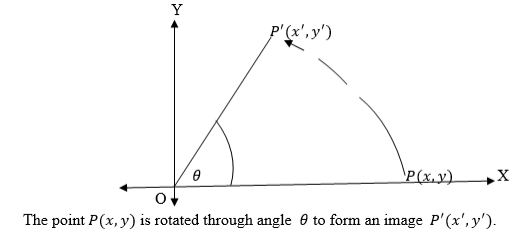Rotation is an isometric mapping and it is usually denoted by R.
Therefore Rθ means rotation of an object through an angleθ.
In
the xy plane, whenθismeasured in the clockwise direction it is negative
and when it is measured in the anticlockwise direction it is positive.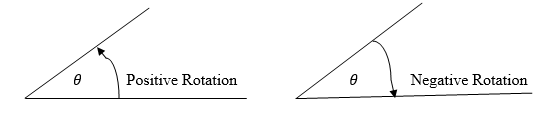Example 31
Find the image of the point P(1,0) after a rotation through 900 about the origin in the anti clockwise direction.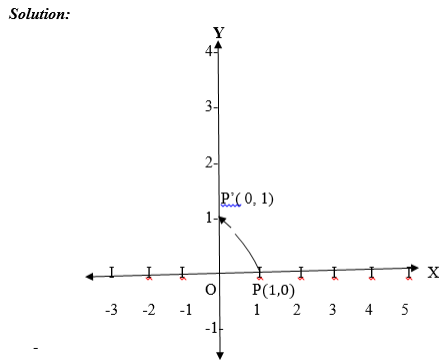P is on the x – axis, so after rotation through 900
about the origin it will be on the y – axis. Since P is 1unit from O,
P¢ is also 1 unit from O, the coordinates of P¢ (0,1) are P¢ (0,1).
Therefore R 900(1,0) = (0,1).
Example 32
Find the image of the point B (4,2) after a rotation through 900 about the origin in the anticlockwise direction.
Solution;
Consider the following figure,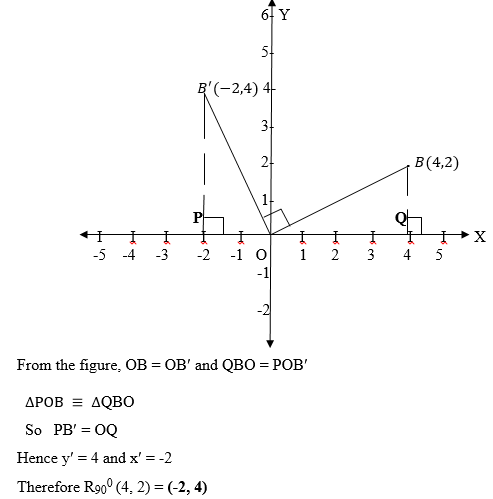Exercise 6
Find the matrix of rotation through
1. 900 about the origin
2. 450 about the origin
3. 270about the origin
Find the image of the point (1,2) under rotation through 1800 ant –clockwise about the origin.
Find the image of the point (-2,1) under rotation through 2700 clockwise about the origin
Find the image of (1,2) after rotation of -900.
Find the image of the line passing through points a (-2,3) and B(2,8) after rotation through 900 clockwise about the origin
General formula for rotation
Consider the following sketch,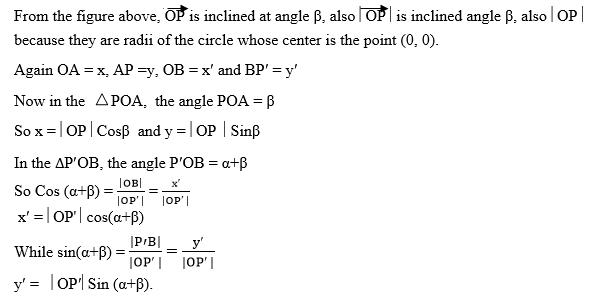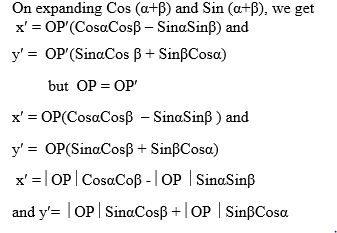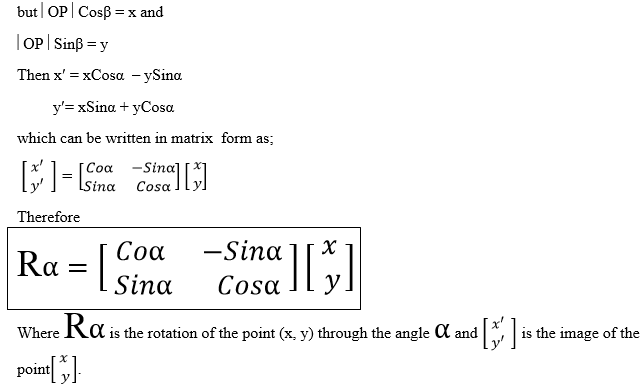Example 33
Find the image of the point (1,2) under a rotation through 1800 anticlockwise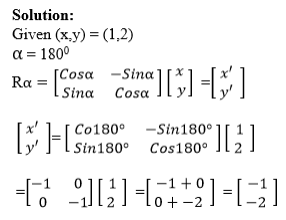Therefore the image of (1, 2) after rotation through 1800 anticlockwise is (-1,-2).
Example 34
Find the image of the point (5,2) under rotation of 900 followed by another rotation of 1800 anticlockwise.
Solution: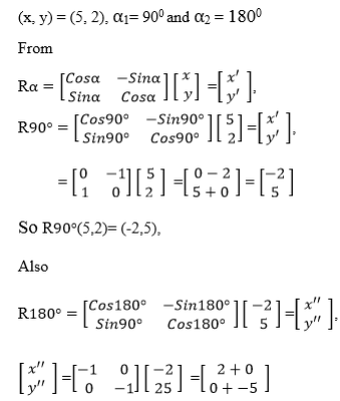Therefore the image of (5,2) under rotation of 90followed by another rotation of 180anticlockwise is (2,-5) .
Translation
Definition:
A translation is a mapping of a point P (x, y) into P’ (x’, y’) by the
Vector (a, b) such that (x’, y’) = (x, y) + (a, b), translation is
denoted by the letter T. So T maps a point (x, y) into x’, y’)
Where (x’, y’) = (x, y) + (a, b)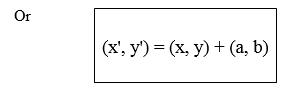Consider
the triangle OPQ whose vertices are (0,0), (3,1) and (3,0) respectively
which is mapped into triangle O¢P¢Q¢ by moving it 2 units in the
positive x direction and 3 units in the positive y direction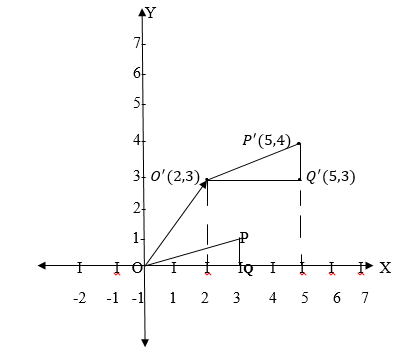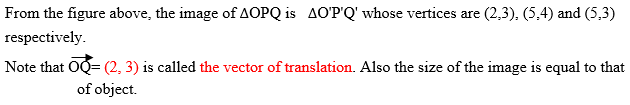Example 35
If T is a translation by the vector (4,3), find the image of (1, 2) under this translation.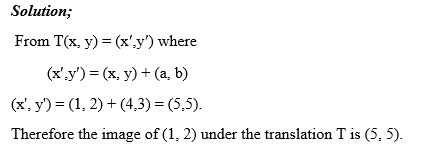Example 36
A translation T maps the point (-3, 2) into (4, 3). Find where (a) T maps the origin (b) T maps the point (7, 4).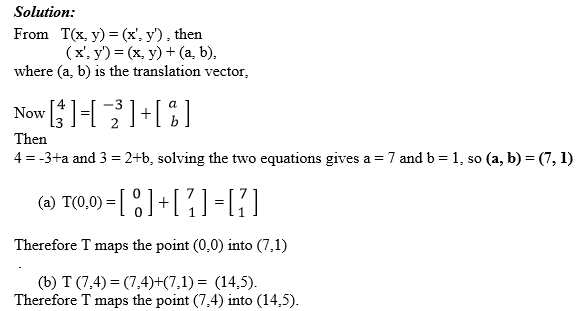Example 37
Find the translation vector which maps the point (6,-6) into (7,16).
Solution
Given that (x, y) = (6,-6) and (x¢, y¢) = (7,16), (a, b) =?
From T (x, y) = (x, y) + (a, b) = (x’, y’),
then (7,16) = (6,-6)+(a,b) which means a=7-6 = 1 and b=16+6 = 22. Therefore translation vector (a,b) = (1,22).
The Enlargement Matrix E in Enlarging Figures
Use the enlargement matrix E in enlarging figures
Definition:
Enlargement is the transformation which magnifies an object such that
its image is proportionally increases on decreased in size by some
factor k. The general matrix of enlargement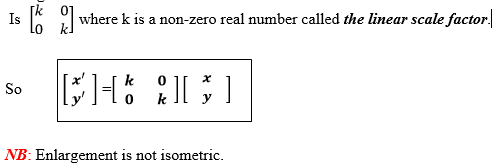Example 38
Find the image of the square with vertices O(0,0), A (1,0), B (1,1) and C (0,1) under the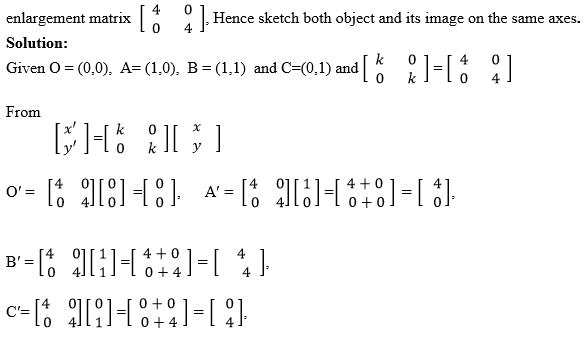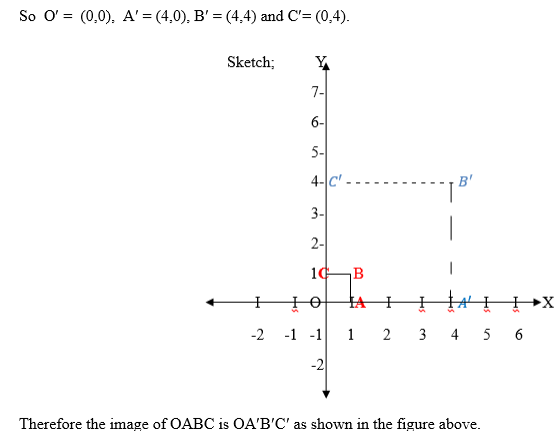Example 39
Find the image of (6, 9) under enlargement by the matrix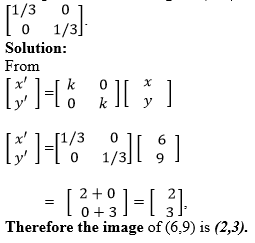Example 40
Draw the image of a unit circle with center O (0,0) under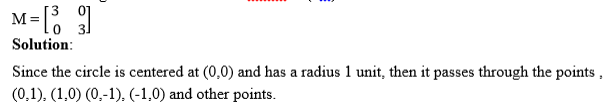Now
the images of these points are (0,3), (3,0), (0,-3), (-3,0) and other
points respectively, where the centre remains (0,0) and the radius
becomes 3 units.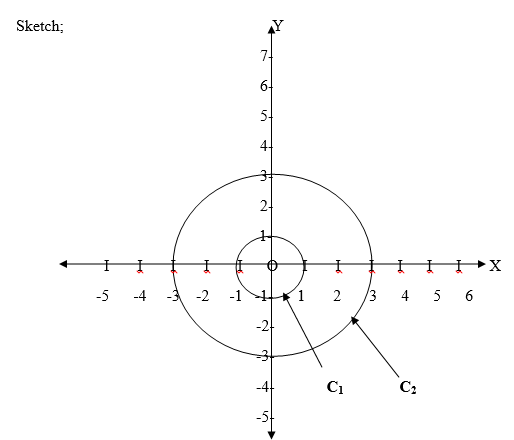I n the figure above, the circle with radius 1 unit and its image with radius 3 units C1 and C2 respectively are shown.
Linear Transformation:
Definition:
For any transformation T, any two vectors U and V and any real number t, T is said to be a linear transformation if and only if
T(t U) = tT(U) and T (U+V) = T(U) + T(V)
Example 41
Show that the rotation by 900about O(0,0) is a linear trans formation
Solution
Let U=(U1,U2) and V =(V1 , V2) be any two vectors in the plane and t be any real number
To show that R900 is the linear transformation we must show that
R900 (tU)= t R900 (U) and
R900 (U + V) = R900 (U) + R900 (V)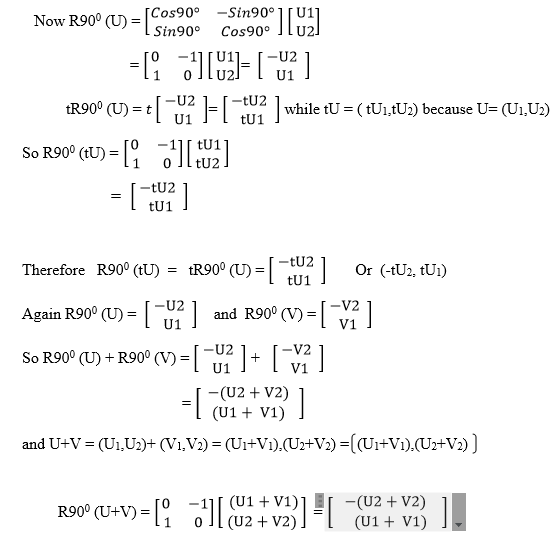Therefore, since R900 (U) + R900 (V) = R900 (U+V) and R900 (tU)= t R900 (U), then R900 is a linear trans formation.
Example 42
Suppose that T is a linear transformation such that
T(U) = (1,-2), T(V) = (-3,-1) for any vectors U and V, find
(a) T(U+ V) (b) T(8U) (c) T(3U -2V)
Solution
(a)Since T is a linear Transformation then
T( U+ V) = T(U) + T(V)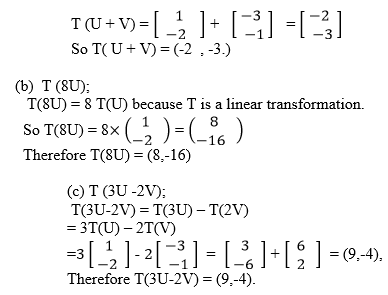Exercise 7
1. If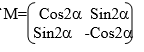2. Is the matrix of reflection in a line inclined at angle a, U=(6,1) , V=(-1,4) and a13500, find (a) m(U+V) (b) m(2V)
If U =(2,-7) and V=(2,-3), find the matrix of linear transformation T such that T(2U)=(-4,14) and T(3V) = (6,9)
4. What is the image of (1,2) under the transformation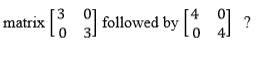5. Given that I is the identify transformation such that I(U) =U for any Vector U, prove that I is a linear transformation.

TOPIC 7: GEOMETRIC AND TRANSFORMATIONS ~ MATHEMATICS FORM 2